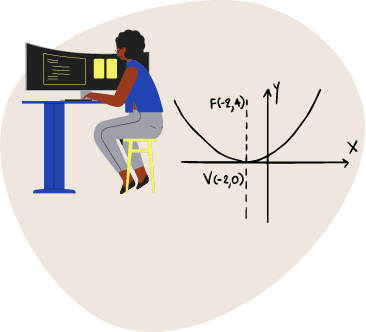# Math for Data Science Visually Explained\$49

To become a Data Scientist, it is crucial to have a strong foundation in Calculus and Linear Algebra. This knowledge enables you to comprehend algorithms thoroughly and you will able to fine-tune them with confidence.

However, understanding mathematical concepts in Data Science can be difficult. In this course, we offer in-depth explanations, including images, diagrams, and analogies, to help you grasp complex ideas.

We will use Pandas and NumPy to apply the concepts.

Pre-order the course to get 10% off.

#### What you’ll learn

• Understand Calculus
• Understand Linear Algebra
##### WHO THIS COURSE IS FOR

This course is for whoever wants to understand the fundamental concepts of Mathematics for Data Science and is willing to learn Data Science concepts.